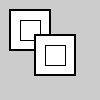# Py5Graphics.push_matrix()#

Pushes the current transformation matrix onto the matrix stack.

## Examples#def setup():
py5.size(100, 100, py5.P2D)

g = py5.create_graphics(60, 60, py5.P2D)
with g.begin_draw():
g.translate(30, 30)
with g.push_matrix():
g.scale(2)
with g.begin_closed_shape():
g.vertex(-10, -10)
g.vertex(10, -10)
g.vertex(10, 10)
g.vertex(-10, 10)
with g.begin_closed_shape():
g.vertex(-10, -10)
g.vertex(10, -10)
g.vertex(10, 10)
g.vertex(-10, 10)

py5.image(g, 0, 0)
py5.image(g, 25, 25)


## Description#

Pushes the current transformation matrix onto the matrix stack. Understanding push_matrix() and Py5Graphics.pop_matrix() requires understanding the concept of a matrix stack. The push_matrix() function saves the current coordinate system to the stack and Py5Graphics.pop_matrix() restores the prior coordinate system. push_matrix() and Py5Graphics.pop_matrix() are used in conjuction with the other transformation functions and may be embedded to control the scope of the transformations.

This method can be used as a context manager to ensure that Py5Graphics.pop_matrix() always gets called, as shown in the example.

This method is the same as push_matrix() but linked to a Py5Graphics object. To see example code for how it can be used, see push_matrix().

Underlying Processing method: PGraphics.pushMatrix

## Signatures#

push_matrix() -> None


Updated on March 06, 2023 02:49:26am UTC